## Famous Mathematicians

Many of the mathematical concepts that we use today were once unknown. Had it not been for famous mathematicians and their contributions, some of those concepts may not be around today. Important thinkers throughout history like Archimedes, Pythagoras, and Benjamin Banneker have helped us understand our world through mathematics and numbers. Read more about the ones mentioned and others below as they are organized in chronological order for your convenience.

Mathematicians of Antiquity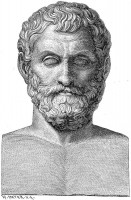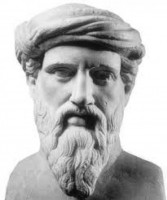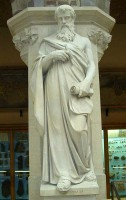Thales (c.624 BC-c.546 BC)
Nationality: Greek
Known for: Organizing and formulating the five theorems of geometry
By using his knowledge of geometry, Thales was able to determine how far a ship was out at sea and he could measure the height of the pyramids based on the lenth of their shadows. He has also received credit for formulating five theorems of geometry.
Pythagoras (c.570 BC-c.495 BC)
Nationality: Greek
Known for: Pythagorean Theorem
Pythagoras is best known for the theorem that is named after him, which helps solve the length of the hypotenuse in a right triangle. He is also credited with creating the tetractys, which are triangular figures that have four rows and each row adds up to ten.
Euclid (fl. 3rd Century BC)
Nationality: Greek
Known for: Euclidean Geometry
Euclid produced a work entitled Euclid’s Elements which was a discussion of all the mathematical principles of his time. It served as a basis for geometry and it is still used in schools today.The work was comprised of 13 volumes and it included number theories, deductions, and other mathematical concepts.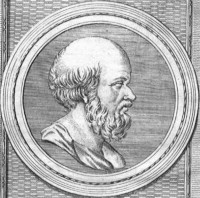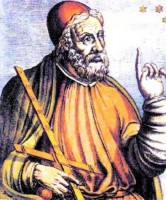Archimedes (c.287 BC-c.212 BC)
Nationality: Greek
Known for: Calculated pi to one of the most accurate figures of his time.
There are several contributions that Archimedes has made to mathematics. One major discovery that he made was a way for finding the volume of a sphere. He also discovered the principal of buoyancy (allegedly while he was taking a bath one evening) and the theory of the Powers of Ten.
Eratosthenes (c.276 BC-c.195 BC)
Nationality: Greek
Known for: First to calculate the earth’s circumference using stades
This Greek mathematician made many accurate calculations for the time in which he lived. He calculated the tilt of the earth on its axis as well as the distance of the earth from the sun. His findings led him to suggest that there should be a “leap day” every four years.
Ptolemy (c.90-c.168)
Nationality: Greco-Roman
Known for: Published Almagest, which applies mathematical theories to the motion of the planets and sun
Although much of his focus was on astronomy, Ptolemy contributed to the field of mathematics in significant ways. One of his major contributions was his table of chord lengths in a circle which remains one of the earliest tables of a trigonometric function today. He also applied ideas of spherical geometry to his study of astronomy.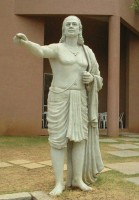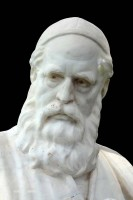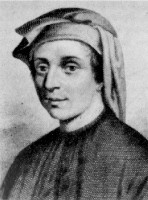Aryabhata (476-550)
Nationality: Indian
Known for: One of the first mathematicians to calculate an approximation of pi
Aryabhatta was one of the best known Indian mathematicians of ancient times. He developed formulas for determining the areas of circles and triangles and he also created the precursor to the table of Sines. He was also the first to use a zero as a placeholder in his place-value system.
Omar Khayyam (1048-1131)
Nationality: Persian
Known for: Treatise on Demonstration of Problems of Algebra
Khayyam helped lay the groundwork for the field of algebra. He also developed methods for sloving cubic equations. His ideas also helped lead the way to developing non-Euclidean geometry.
Fibonacci (c.1170-c.1250)
Nationality: Italian
Known for: Fibonacci Numbers
Also known as Fibonacci, Leonardo Bigollo was mainly responsible for educating Europeans about the Hindu-Arabic numeral system by using one of his writings – Book of Calculation. He also started Fibonacci numbers, which is a sequence of numbers in which each number is the sum of the two numbers preceding it.

Mathematicians of the 1400s-1600s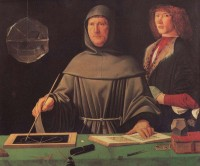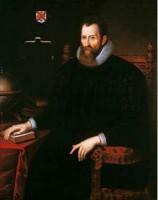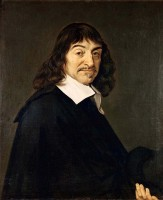Luca Pacioli (1445-1517)
Nationality: Italian
Known for: The Father of Accounting
Pacioli published a textbook entitled Everything about Arithmetic, Geometry and Proportion in 1494. He also spend much of his time translating Elements, Euclid’s major work, into Latin. He is also credited with creating the first ledger and journal system for bookkeeping which revolutionized the accounting industry.
John Napier (1550-1617)
Nationality: Scottish
Known for: Discovered logarithms
In his work Mirifici Logarithmorum Canonis Descriptio, Napier included nearly 150 pages related to natural logarithms and tables related to those humbers. He also included theories regarding spherical trigonometry, which is also known as Napier’s Rules of Circular Parts.
Rene Descartes (1596-1650)
Nationality: French
Known for: Cartesian Coordinate System
Descartes has made several contributions to the field of mathematics. In addition to the Cartesian coordinate system, he is also referred to as the father of analytical geometry. Descartes was also the first to use superscripts to denote exponents and he began the custom of using letters to represent unknown numbers in algebraic equations.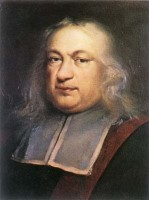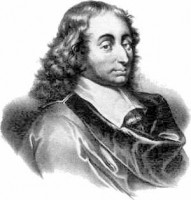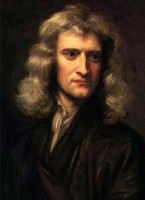Pierre de Fermat (c.1607-1665)
Nationality: French
Known for: Fermat’s Last Theorem
Fermat was beginning his work in analytical geometry before Descartes published his works on the topic. He was also the first mathematician to evaluate integral power functions and he also studied Pell’s equation. Fermat numbers were also named after him.
Blaise Pascal (1623-1662)
Nationality: French
Known for: Pascal’s Calculators
Pascal was a pioneer in the field of mathematics. He helped create the field of projective geometry when he was only a teenager and he helped influence probability theory by corresponding with Pierre de Fermat, a fellow mathematician.
Isaac Newton (1642-1727)
Nationality: English
Known for: Newton’s method for estimating roots of a function
Newton is credited with being one of the developers of infinitesimal calculus, although Gottfried Leibniz has disputed this. Some of the other things that Newton is known for creating include the generalized binomial theorem, Newton’s identities, and more.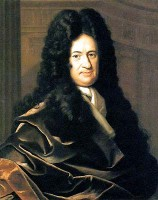Gottfried Leibniz (1646-1716)
Nationality: German
Known for: Helped refine the binary systems which became the foundation for today’s computers
Along with Isaac Newton, Leibniz helped create the field of infinitesimal calculus, although there is some controversy concerning how large Leibniz’s role was in the discovery. He was also the first to notice that coefficients of a linear equation could be manipulated into a matrix to find if there is a solution.

Mathematicians of the 1700s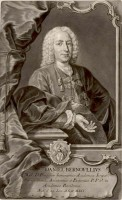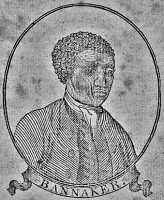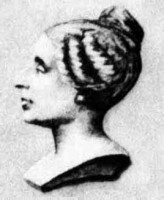Daniel Bernoulli (1700-1782)
Nationality: Swiss
Known for: Formulated a way to measure blood pressure; Bernoulli Principle
In 1724, Bernoulli published Mathematical Exercises, his first book regarding marth. In addition, he discovered the relationship between the flow of blood compared to the amount of pressure that it is under. Bernoulli is also credited with discovering the fluid equation and the Bernoulli Principle, which is used in aerodynamics.
Benjamin Banneker (1731-1806)
Nationality: African American
Known for: Developed a mathematical formula to predict a solar eclipse
As a freed slave in the 18th century, Banneker is considered the first African American scientist. But his interest in mathematics and astronomy helped bring him recognition. He is credited with publishing several state almanacs with tide tables, the times for future eclipses and formulas for medicines.
Sophie Germain (1776-1831)
Nationality: French
Known for: Formulated Sophie Germain’s theorem
Germain’s main focus was in number theory. She was inspired by reading the works of other mathematicians, including Gauss and Legendre. She also presented a derivation of a differential equation which won her a prize from the Paris Academy of Sciences. Her work with Fermat’s Last Theorem helped bring her even more recognition.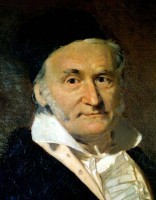Carl Gauss (1777-1855)
Nationality: German
Known for: Often referred to as the Prince of Mathematicians
After creating a 17-sided polygon, which was thought impossible by most people of his time, Gauss decided to delve into the field of mathematics. He wrote a book about number theory and also wrote Disquisitines Arithmeticae, which was one of the most influential math books in history.

Mathematicians of the 1800s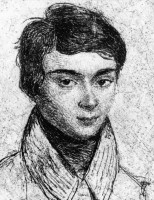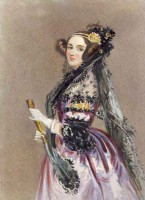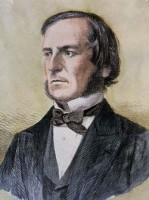Evariste Galois (1811-1832)
Nationality: French
Known for: Helped lay the foundations for Galois theory and group theory
Solved a long-term problem that had perplexed mathematicians for years by determining a way to solve polynomials by using radicals. He is also credited with being the first person to use the term “group” in the field of mathematics when referring to a group of permutations.
Nationality: English
Known for: Referred to as the world’s first computer programmer
Lovelace worked closely with Charles Babbage, the man credited with creating the first mechanical computer. She helped write instructions for using the computer, which earned her the reputation of the first computer programmer in the world. She also translated articles about the machine for publication in magazines around the world.
George Boole (1815-1864)
Nationality: English
Known for: Author of The Laws of Thought; also invented Boolean logic
In some ways, Boole is considered to be the founder of the computer science field because his theories and contributions to mathematics helped lay the groundwork for digital computers. Boolean logic, for instance, was fundamental in developing computer science and abstractly describing digital circuits.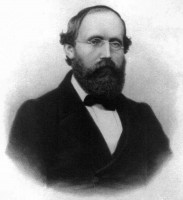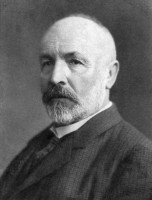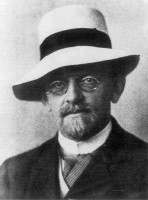Bernhard Riemann (1826-1866)
Nationality: German
Known for: Developed Riemannian geometry
Riemann’s work in geometry helped lay the groundwork for topology, a field of modern mathematical physics that is still around today. At the time of his death, he was working on a project regarding a collection of numbers and points throughout space. He also developed the Riemann integral and the Riemann zeta function.
Georg Cantor (1845-1918)
Nationality: German
Known for: Formulated the set theory
In 1889, following a nervous breakdown, Cantor created the German Mathematical Society and worked on creating the first International Congress of Mathematicians. He published a paper dealith with algebraic numbers and proving that they can be corresponded to positive integers with both having the same power.
David Hilbert (1862-1943)
Nationality: German
Known for: Formulated Hilbert Spaces, a major theory in functional analysis
As one of the founders of proof theory, Hilbert has been referred to as one of the most influential mathematicians in the last couple centuries. He has also helped make a distinction between mathematics and metamathematics, which is the study of using mathematics with mathematical methods.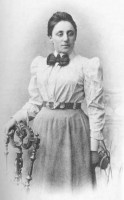Emmy Noether (1882-1935)
Nationality: German
Known for: Formulated Noether’s theorem
One of Noether’s concentrations in the field of mathematics was mathematical rings. She made several breakthroughs in abstract algebra and her paper – Theory of Ideals in Ring Domains – became the groundwork for ring theory. In 1932, she won the Ackermann-teubner Memorial Prize in Mathematics for her contributions.

Mathematicians of the 1900s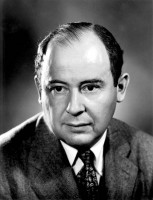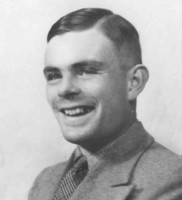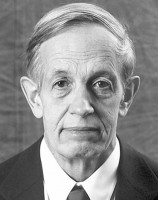John von Neumann (1903-1957)
Nationality: Hungarian
Known for: Established Dirac-von Neuman axioms, the mathematical foundation for quantum mechanics
Von Neumann contributed greatly to the field of mathematics. In addition to laying the groundwork for quantum mechanics, he had dozens of publications, including Mathematical Foundations of Quantum Mechanics and Computer and the Brain. He also applied mathematics to the field of nuclear energy when working on the Manhattan Project.
Alan Turing (1912-1954)
Nationality: British
Known for: Using computation theory to create the Turing machine
As one of the most respected mathematicians and logicians, Turing made great strides in these fields. He helped develop the field of mathematical biology and he published a paper about the topic entitled The Chemical Basis of Morphogenesis. He also worked with algorithms and computations to design the first general purpose computer.
John Nash (born 1928)
Nationality: American
Known for: Nash equilibrium
Nash focused on algebraic geometry in his mathematical studies. He developed the Nash embedding theorem which demonstrated abstract Riemannian manifolds could be isometrically attained as a Euclidean space submanifold. He also contributed ideas to singularity theory and parabolic partial differential equations.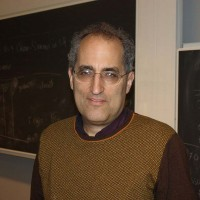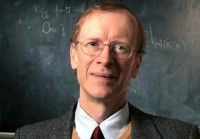Edward Witten (born 1951)
Nationality: American
Known for: Proposed the M-theory of the universe
Witten has published more than 300 works that focus on mathematical topics like string theory, geology, topology and quantum field theory. He has won prizes for his work in string theory, including the Fields Medal in 1990 and the Dirac medal from the International Center for Theoretical Physics in 1985.
Andrew Wiles (born 1953)
Nationality: British
Known for: Proved Fermat’s Last Theorem
Beginning in his childhood years, Wiles was fascinated with Fermat’s Last Theorem. He spent nearly six years working on proving the theorem. He has appeared on the BBC for his work and he has won several prizes in his career, including the Fermat Prize in 1995 and a Royal Medal in 1996.